Salish Sea NEMO Bathymetry¶

This notebook documents the bathymetry used for the Salish Sea NEMO runs.

The first part of the notebook explores and plots the bathymetry.

In :
%matplotlib inline
import matplotlib.pyplot as plt
import netCDF4 as nc
import numpy as np
from salishsea_tools import nc_tools

bathy_tools is a collection of Python functions that were developed to encapsulate the details of working with a bathymetry netCDF file.

In :
from salishsea_tools import bathy_tools

Open the file and get some basic information about it. The r mode shown opens the file in read-only mode. Use r+ as the mode if you want to change the bathymetry.

In :
bathy = nc.Dataset('../../NEMO-forcing/grid/bathy_meter_SalishSea.nc', 'r')
print bathy.file_format
bathy_tools.show_dimensions(bathy)
bathy_tools.show_variables(bathy)
NETCDF4
<type 'netCDF4.Dimension'>: name = 'y', size = 898

<type 'netCDF4.Dimension'>: name = 'x', size = 398

[u'nav_lon', u'nav_lat', u'Bathymetry']

Assign short names to the variables and get some basic information about their values:

In :
lats = bathy.variables['nav_lat']
lons = bathy.variables['nav_lon']
depths = bathy.variables['Bathymetry']
print lats
print lons
print depths
<type 'netCDF4.Variable'>
float64 nav_lat(y, x)
units: degrees north
valid_range: [ 46.85966492  51.10480118]
unlimited dimensions:
current shape = (898, 398)

<type 'netCDF4.Variable'>
float64 nav_lon(y, x)
units: degrees east
valid_range: [-126.40029144 -121.31835175]
unlimited dimensions:
current shape = (898, 398)

<type 'netCDF4.Variable'>
float64 Bathymetry(y, x)
_FillValue: 0.0
least_significant_digit: 1
units: m
valid_range: [   0.  428.]
unlimited dimensions:
current shape = (898, 398)

In :
lat_stats = bathy_tools.min_mid_max(lats)
lon_stats = bathy_tools.min_mid_max(lons)
print lat_stats
print lon_stats
print np.min(depths), np.max(depths)
(46.859664916992188, 49.00848388671875, 51.104801177978516)
(-126.40029144287109, -123.86368560791016, -121.31835174560547)
0.0 428.0

Plot a colour-mesh plot of the whole dataset. Use fig.savefig('filename.png') to store the plot as an image file in the pwd.

In :
fig = bathy_tools.plot_colourmesh(
bathy, 'Salish Sea Bathymetry',
axis_limits=(-126.3, -122.15, 47.05, 51))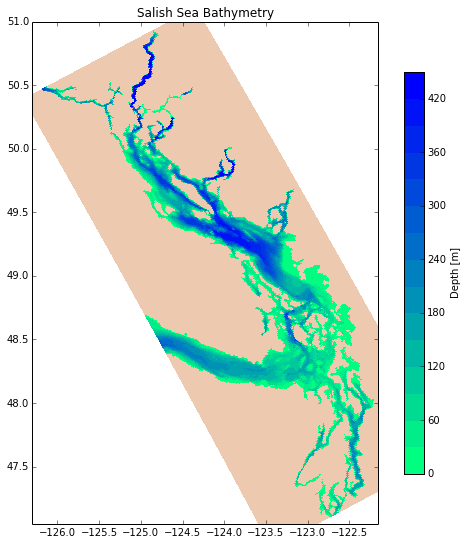Conversion to netCDF4¶

The variable attributes, and the variable values were copied to a new netCDF4 dataset with zlib compression enabled for all variables and least_significant_digit=1 set for the depth values. No global attributes were found in the netCDF3 version of the file.

The resulting file is 545kb in contrast to 4.1Mb as a netCDF3_CLASSIC file. The netCDF3 file was replaced in the NEMO-forcing repo with the new netCDF4 one.

The code cells that were run are shown below as syntax highlighted text because they only need to be run once.

nc4_bathy = nc.Dataset('../../NEMO-forcing/grid/bathy_meter_SalishSea_nc4.nc', 'w')

Dimensions:

nc4_bathy.createDimension('y', 898)
nc4_bathy.createDimension('x', 398)
bathy_tools.show_dimensions(nc4_bathy)

Variables and their attributes.

Note that zlib=True for all variables and least significant_digit and fill_value are set for the depths.

lons = nc4_bathy.createVariable('nav_lon', float, ('y', 'x'), zlib=True)
lons.units = 'degrees east'
lats = nc4_bathy.createVariable('nav_lat', float, ('y', 'x'), zlib=True)
lats.units = 'degrees north'
depths = nc4_bathy.createVariable(
'Bathymetry', float, ('y', 'x'),
zlib=True, least_significant_digit=1, fill_value=0)
depths.units = 'm'
bathy_tools.show_variables(nc4_bathy)
print lons
print lats
print depths

Copy data values from netCDF3 dataset to our new one:

lons[:] = bathy.variables['nav_lon']
lats[:] = bathy.variables['nav_lat']
depths[:] = bathy.variables['Bathymetry']

Verify the new dataset, and set valid_range attributes for latitude and longitude:

lat_stats = bathy_tools.min_mid_max(lats)
lats.valid_range = np.array((lat_stats, lat_stats))
lon_stats = bathy_tools.min_mid_max(lons)
lons.valid_range = np.array((lon_stats, lon_stats))
print lat_stats
print lon_stats
print np.min(depths), np.max(depths)

Commit the new dataset to disk:

nc4_bathy.close()

Depth Clipping¶

bathy = nc.Dataset('../../NEMO-forcing/grid/bathy_meter_SalishSea.nc', 'r+')
depths = bathy.variables['Bathymetry']

Mask all depths less than 4m so that the minimum model depth is 4m.

In :
shallow = np.logical_and(depths[:] > 0, depths[:] < 4)
dep = depths[:]
dep[shallow] = 4
depths[:] = dep

Test that the main body of the Strait of Georgia, maximum depth is 428 m. Do this by brute force, plotting with circles anywhere the depth is greater than 428m. Note that this takes a long time to run, so ask yourself: do I really need to rerun this.

In :
def plot_circles():
for i in range(0,898):
for j in range(0,398):
if depths[i,j] > 428:
plt.plot(lons[i,j],lats[i,j],'ko')
return
In :
fig = bathy_tools.plot_colourmesh(
bathy, 'Salish Sea Bathymetry',
axis_limits=(-126.3, -122.15, 47.05, 51))
plot_circles()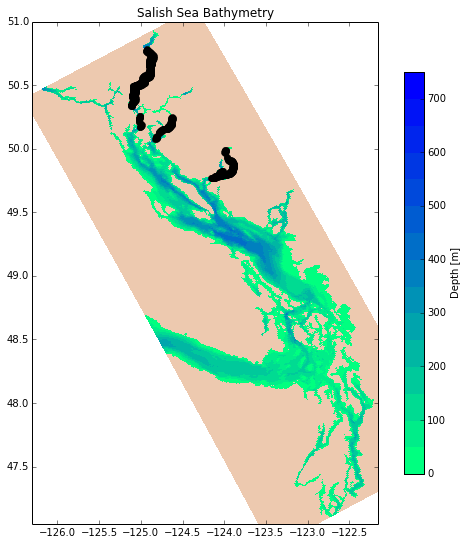Mask all depths greater than 428m so that the maximum model depth is 428m.

In :
shallow = depths[:] > 428
dep = depths[:]
dep[shallow] = 428
depths[:] = dep

Set the valid_range attribute of the Bathymetry variable, and commit the dataset to disk.

In :
depths.valid_range = np.array((np.min(depths), np.max(depths)))
print depths
<type 'netCDF4.Variable'>
float64 Bathymetry(y, x)
_FillValue: 0.0
least_significant_digit: 1
units: m
valid_range: [   0.  428.]
unlimited dimensions:
current shape = (898, 398)

bathy.close()

Update netCDF Attributes¶

Subsequent to the creation of the netCDF4 bathymetry file a more extensive standard for attributes was adopted, so it was applied.

nc_filepath = '../../NEMO-forcing/grid/bathy_meter_SalishSea.nc'
bathy = nc.Dataset(nc_filepath, 'r+')
nc_tools.show_dataset_attrs(bathy)
In :
nc_tools.init_dataset_attrs(bathy, 'Salish Sea NEMO Bathymetry', 'SalishSeaBathy', nc_filepath)
file format: NETCDF4
Conventions: CF-1.6
title: Salish Sea NEMO Bathymetry
institution: Dept of Earth, Ocean & Atmospheric Sciences, University of British Columbia
source: https://bitbucket.org/salishsea/tools/src/tip/bathymetry/SalishSeaBathy.ipynb
references: https://bitbucket.org/salishsea/nemo-forcing/src/tip/grid/bathy_meter_SalishSea.nc
history: [2013-11-21 20:05:06] Created netCDF4 zlib=True dataset.
comment:
In :
bathy.comment = 'Based on 1_bathymetry_seagrid_WestCoast.nc file from 2-Oct-2013 WCSD_PREP tarball provided by J-P Paquin.'
In :
nc_tools.show_variable_attrs(bathy)
<type 'netCDF4.Variable'>
float64 nav_lon(y, x)
units: degrees east
valid_range: [-126.40029144 -121.31835175]
unlimited dimensions:
current shape = (898, 398)

<type 'netCDF4.Variable'>
float64 nav_lat(y, x)
units: degrees north
valid_range: [ 46.85966492  51.10480118]
unlimited dimensions:
current shape = (898, 398)

<type 'netCDF4.Variable'>
float64 Bathymetry(y, x)
_FillValue: 0.0
least_significant_digit: 1
units: m
valid_range: [   0.  428.]
unlimited dimensions:
current shape = (898, 398)

In :
bathy.variables['nav_lon'].long_name = 'Longitude'
bathy.variables['nav_lat'].long_name = 'Latitude'
bathy.variables['Bathymetry'].long_name = 'Depth'
bathy.variables['Bathymetry'].positive = 'down'
In :
nc_tools.show_variable_attrs(bathy)
<type 'netCDF4.Variable'>
float64 nav_lon(y, x)
units: degrees east
valid_range: [-126.40029144 -121.31835175]
long_name: Longitude
unlimited dimensions:
current shape = (898, 398)

<type 'netCDF4.Variable'>
float64 nav_lat(y, x)
units: degrees north
valid_range: [ 46.85966492  51.10480118]
long_name: Latitude
unlimited dimensions:
current shape = (898, 398)

<type 'netCDF4.Variable'>
float64 Bathymetry(y, x)
_FillValue: 0.0
least_significant_digit: 1
units: m
valid_range: [   0.  428.]
long_name: Depth
positive: down
unlimited dimensions:
current shape = (898, 398)

In :
nc_tools.check_dataset_attrs(bathy)
In :
bathy.history = """
[2013-10-30 13:18] Created netCDF4 zlib=True dataset.
[2013-10-30 15:22] Set depths between 0 and 4m to 4m and those >428m to 428m.
[2013-10-31 17:10] Algorithmic smoothing.
[2013-11-21 20:14] Updated dataset and variable attributes to CF-1.6 conventions & project standards.
"""
bathy.close()

Remove Bad Bathymetry Regions¶

After the bathymetry had been in use for several runs it was noted that an area at the east end of Jervis Inlet and another region around Toba Inlet were anomalously shallow. The source of the incorrect depths in those area was traced back to the Cascadian bathymetry data that was used as the source for our bathymetry. Since the 2 regions in question are not very significant in the overall Salish Sea it was decided to remove them from the model bathymetry by setting their depths to zero until correct bathymetry source data can be obtained.

The bathymetry file was reverted to changeset 3b301b5b9b6d to remove the algorithmic smoothing before applying the following modifications.

nc_filepath = '../../NEMO-forcing/grid/bathy_meter_SalishSea.nc'
bathy = nc.Dataset(nc_filepath, 'r+')
depths = bathy.variables['Bathymetry']
In :
def plot_bathy_grid_mesh(title):
plt.figure(figsize=(14, 14))
bathy_tools.set_aspect_ratio(bathy.variables['nav_lat'])
plt.pcolormesh(depths[:], cmap='winter_r')
plt.colorbar()
plt.title(title)
In :
plot_bathy_grid_mesh('Before Modifications')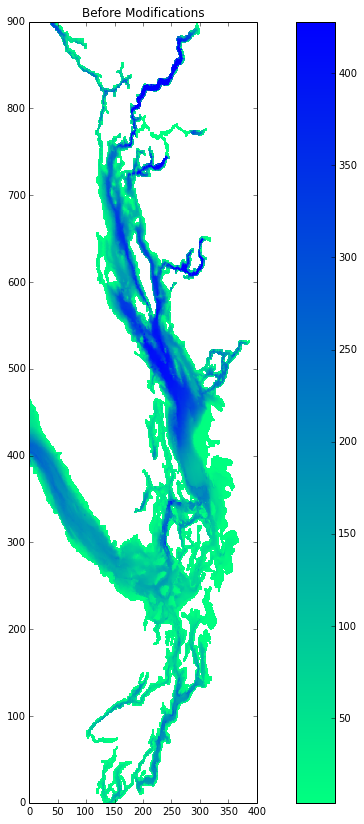In :
depths = bathy_tools.zero_jervis_end(depths)
In :
plot_bathy_grid_mesh('Jervis Inlet End Zeroed')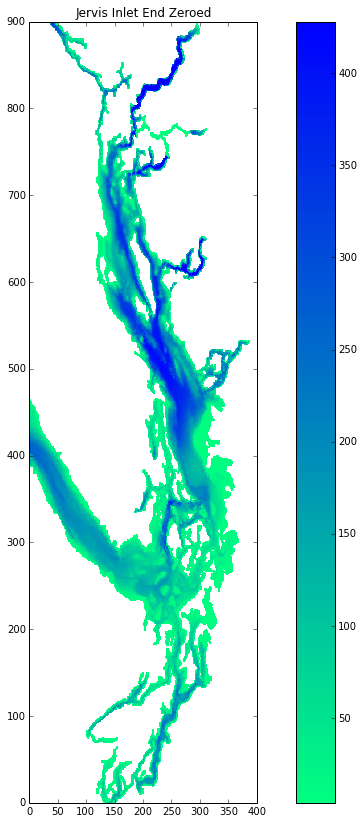In :
depths = bathy_tools.zero_toba_region(depths)
In :
plot_bathy_grid_mesh('Toba Inlet Region Zeroed')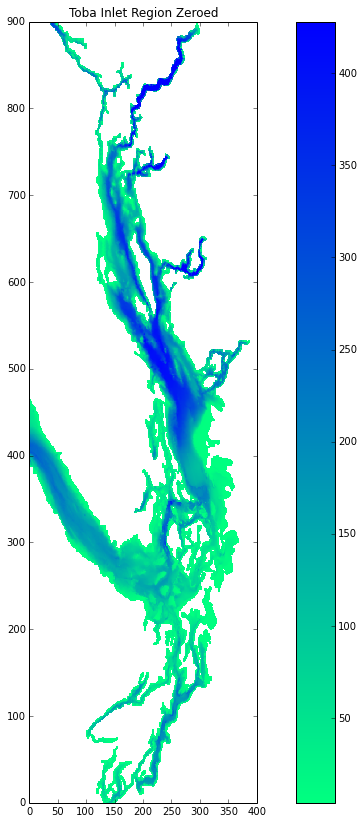In :
bathy.history += """
[2013-11-21 20:47] Removed east end of Jervis Inlet and Toba Inlet region due to deficient source bathymetry data in Cascadia dataset.
"""
In :
bathy.history
Out:
u'\n    [2013-10-30 13:18] Created netCDF4 zlib=True dataset.\n    [2013-10-30 15:22] Set depths between 0 and 4m to 4m and those >428m to 428m.\n    [2013-10-31 17:10] Algorithmic smoothing.\n    [2013-11-21 20:14] Updated dataset and variable attributes to CF-1.6 conventions & project standards.\n\n    [2013-11-21 20:47] Removed east end of Jervis Inlet and Toba Inlet region due to deficient source bathymetry data in Cascadia dataset.\n'
In :
nc_tools.check_dataset_attrs(bathy)
bathy.close()

Algorithmic Smoothing¶

Susan developed a smoothing algorithm that successively adjusts the depths at adjacent grid point for pairs of grid points that have the greatest normalized difference in depth. The normalization factor is the average depth at the pair of grid points. The pair by pair adjustment of grid points is repeated until the maximum normalized depth difference has dropped below a threshold.

The bathy_tools.smooth() function used below is the final implementation of the algorithm. Its development can be seen in TowardSmoothing.ipynb notebook.

nc_filepath = '../../NEMO-forcing/grid/bathy_meter_SalishSea.nc'
bathy = nc.Dataset(nc_filepath, 'r+')
depths = bathy.variables['Bathymetry']

Smooth the bathymetry by successively adjusting depths that have the greatest normalized difference until all of those differences are below a threshhold. This takes several minutes...

In :
depths = bathy_tools.smooth(depths, max_norm_depth_diff=0.8, smooth_factor=0.2)

Confirm that the threshold has been achieved.

In :
diffs_lat = bathy_tools.calc_norm_depth_diffs(depths, delta_lat=1, delta_lon=0)
lat_ij = bathy_tools.argmax(diffs_lat)
diffs_lon = bathy_tools.calc_norm_depth_diffs(depths, delta_lat=0, delta_lon=1)
lon_ij = bathy_tools.argmax(diffs_lon)

print diffs_lat[lat_ij], diffs_lon[lon_ij]
0.8 0.8

Compare the smoothed bathymetry to the original.

In :
orig_bathy = nc.Dataset('../../NEMO-forcing/grid/bathy_meter_SalishSea_orig.nc', 'r')
orig_depths = orig_bathy.variables['Bathymetry']
In :
plt.figure(figsize=(14, 14))
diffs = orig_depths[:] - depths[:]
plt.pcolormesh(-diffs, cmap=plt.cm.bwr, vmin=-80, vmax=80)
plt.colorbar()
Out:
<matplotlib.colorbar.Colorbar instance at 0x1056cd710>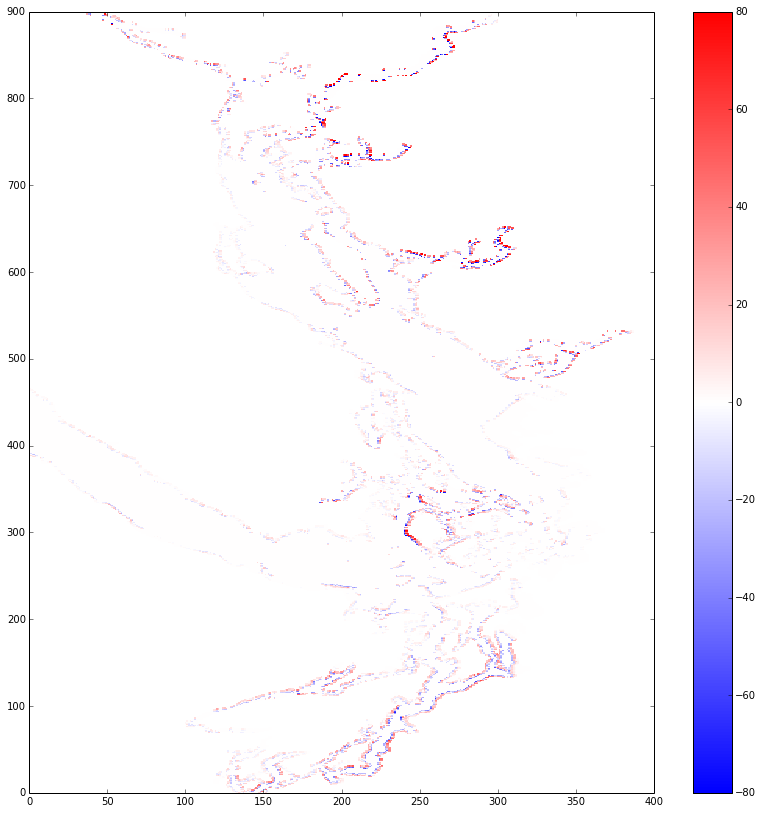In :
np.min(diffs), np.max(diffs)
Out:
(-140.625, 140.625)
In :
fig = bathy_tools.plot_colourmesh(
bathy, 'Salish Sea Bathymetry',
axis_limits=(-126.3, -122.15, 47.05, 51))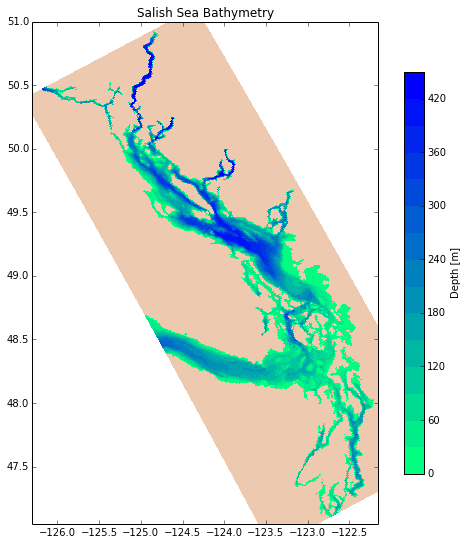In :
bathy.history = """
[2013-10-30 13:18] Created netCDF4 zlib=True dataset.
[2013-10-30 15:22] Set depths between 0 and 4m to 4m and those >428m to 428m.
[2013-10-31 17:10] Algorithmic smoothing.
[2013-11-21 19:53] Reverted to pre-smothing dataset (repo rev 3b301b5b9b6d).
[2013-11-21 20:14] Updated dataset and variable attributes to CF-1.6 conventions & project standards.
[2013-11-21 20:47] Removed east end of Jervis Inlet and Toba Inlet region due to deficient source bathymetry data in Cascadia dataset.
[2013-11-21 21:52] Algorithmic smoothing.
"""
In :
bathy.history
Out:
u'\n    [2013-10-30 13:18] Created netCDF4 zlib=True dataset.\n    [2013-10-30 15:22] Set depths between 0 and 4m to 4m and those >428m to 428m.\n    [2013-10-31 17:10] Algorithmic smoothing.\n    [2013-11-21 19:53] Reverted to pre-smothing dataset (repo rev 3b301b5b9b6d).\n    [2013-11-21 20:14] Updated dataset and variable attributes to CF-1.6 conventions & project standards.\n    [2013-11-21 20:47] Removed east end of Jervis Inlet and Toba Inlet region due to deficient source bathymetry data in Cascadia dataset.\n    [2013-11-21 21:52] Algorithmic smoothing.\n'
In :
nc_tools.check_dataset_attrs(bathy)
bathy.close()
In [ ]: